# Unitary Method

Go back to  'Ratio, Proportion, Percentages'

## Introduction to Unitary Method

Ever wondered if it takes 5 machines to make 5 objects in 5 minutes, how much time will it take 100 machines to make 200 objects?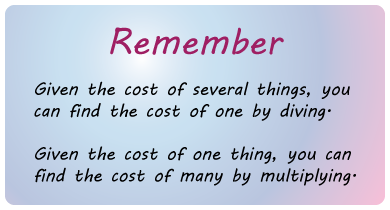## The Big Idea: What is the Unitary method?

The unitary method, in essence, is all about finding the “per unit value”.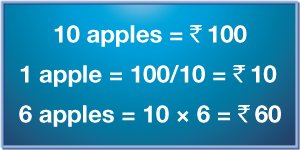This thus becomes important in more advanced ideas like determining the amount of time, resources or capital required for certain types of problems, often which have their own constraints.

Let’s try to answer the Maggi example. So how much time does it take?

Suppose you have invited your friends over for a meal and you need to cook the 7 bowls of Maggi, if you know how much time it takes to make ONE bowl of Maggi, you can optimize your process of cooking to maximize your output. So, HOW DO YOU DO IT?!

The answer lies with the unitary method.

We know:

\begin{align} & 3{\text{ Maggis}}\;\;\; \to \;\;\;12{\text{ minutes}} \hfill \\ & 1{\text{ Maggi}}\;\;\;\,\, \to \;\;\;\frac{{12}}{3} = 4{\text{ Minutes}} \hfill \\ \end{align}

So,

\begin{align}7{\text{ Maggis}}\;\;\; \to \;\;\;4 \times 7 = 28{\text{ Minutes}}\end{align}

Take a look at the illustration below, it highlights a very simple way to understand the calculation involved in the process of finding the per unit value of things: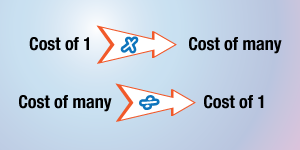## What is the importance of the unitary method?

The unitary method forms an important part of more advanced mathematical concepts such as optimization and operations research where problem statements are converted into equations and then solved.

For example, you go to a supermarket and see two offers on the same product (say sugar) from 2 different brands:

• Brand A is offering 500g sugar for Rs. 80

• Brand B is offering 750g of sugar for Rs. 10

Which brand is the better deal? With Unitary method, your life would be made extremely simple!

The requirements are fed into software with predefined constraints that tells them exactly what needs to be done with the design.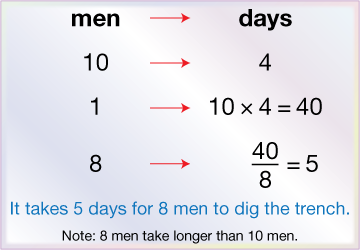Likewise, all grocery store managers who work on limited budgets must decide on what items to buy (and in what quantity) based on constraints like consumer demand and selling time. They again formulate these problem statements into equations and solve to find unknown values graphically.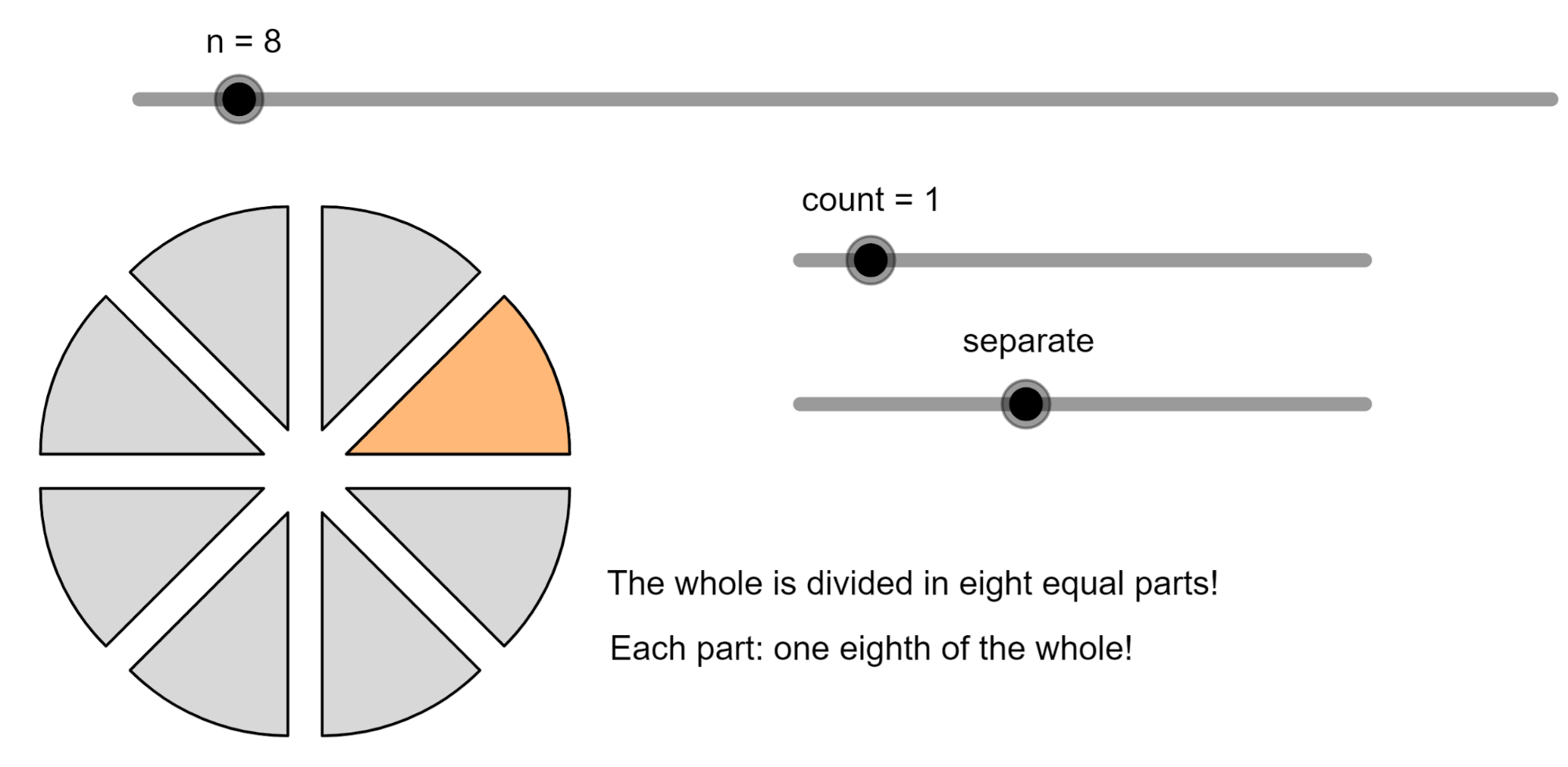### Common doubts regarding the Unitary method

It’s common for students who’ve studied such concepts to find it difficult to tell exactly what needs to be found.

First things first, the idea of the unitary method requires problem statements to be converted into equations so that the required unknown value can be found.

In any problem where items are equated with a certain value such as ”7 books cost Rs.150”, always understand that the problem would require you to either find the books per Rupee or the Rupees per book.

More advanced topics deal with adding quantities for other items (such as pens and pencils in the case above).

The trick is to keep the unit consistent in all the equations to what you’re trying to find. Let’s take the example above for an advanced case.

Suppose you’ve got Rs 100 to spare and you need to find the number of books and pens you could buy given that the price of a book and pen are Rs 5 and Rs 10 respectively. You would thus proceed in the following way:-

(Rs 5/book)*x + (Rs 10/ pen)*y = 100, where x and y are the unknown quantities to be found.

These values would then be graphed on a graph sheet with x and y representing the axes producing a host of values to match Rs 100.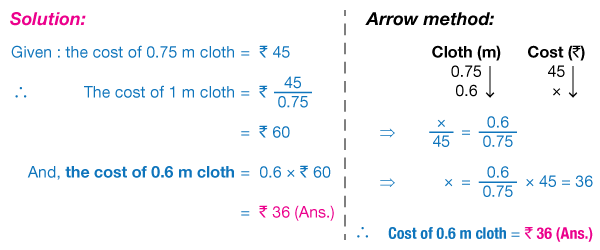Another common issue is the translation of problem statements that don’t use proportions. Certain unitary method constraints can involve values that say that they are less than or greater than other values (for example, 5 workers can paint a wall in less than 4 hours).

In such cases, the equation sign would change and the same arithmetic operations would be performed. To bring these values to a graph, the area over or under a graph would be selected depending on the sign.